Apr 15, 2015

(Advanced) Cheryl's Birthday Puzzle

Source: Sent to me by Prateek Chandra Jha (IIT Bombay)

Problem:
This problem is inspired by the Cheryl's Birthday Puzzle (FB Post, Guardian Link).

Paul, Sam and Dean are assigned the task of figuring out two numbers. They get the following information:

Both numbers are integers between (including) 1 and 1000

Both numbers may also be identical.

Paul is told the product of the two numbers, Sam the sum and Dean the difference. After receiving their number, the following conversation takes place:
Paul: I do not know the two numbers.
Sam: You did not have to tell me that, I already knew that.
Paul: Then I now know the two numbers.
Sam: I also know them.
Dean: I do not know the two numbers. I can only guess one which may probably be correct but I am not sure.
Paul: I know which one you are assuming but it is incorrect.
Dean: Ok, I also know the two numbers.

What are the two numbers?

Disclaimer:
Its not a puzzle for 14-15 year olds like Cheryl's

1.25 and 1 ?

2.Is the answer 64 and 73?

3.I also think that it is probably 64 and 73. I wrote a code to get to that answer, and I would like to know if there are better solutions.

4.5.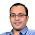6.How did people get 64 and 73? What was the other pair of numbers Dean was considering? It couldn't have been 1 and 10 from what I can see.

7.Here's my code in python (without comments) http://pastebin.com/YfyQ8WUX . It returns 12 pairs of possible solutions, which are admissible until the last 2 statements are made. Of these (64,73) seems most likely, given the last two statements.

8.I am doing something wrong here, because I am finding that there are no numbers which fit into the conversation.

Paula’s first statement implies that the product is not a prime or one – if it’s prime or one, Paula knows the two numbers.

Sue’s first statement implies that the sum is not of the form p+1 or 2, where p is prime - if the sum is of the form p+1 or 2, then Sue wouldn’t know that already.

Paula’s second statement implies that the two numbers are prime – if one of the numbers is not prime, then there are at least two possible sets of numbers.

Sue’s second statement implies that the sum of the numbers is one of the four even numbers that are the sum of a unique pair of two primes 4=2+2, 6=3+3, 8=5+3, 12=7+5. But all of these are of the form p+1, where p is prime.

Hence, there can be no such numbers.

Where is my mistake?

1.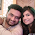product = 8
sum = 9
difference = 7
I thought on similar lines. but i think there can be many sets by the first 4 statements.

9.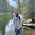@Betlazel - take a look at your first line "Paula's first statement implies..."

-Krishna

10.How is it wrong, Krishna? It should be Paul and Sue should be Sam. I got the puzzle from a different site.

11.64 and 73 can't be the solution for 73 is a prime number and if paul would have got 64*73 he could very well factorise and get the numbers and hence the solution.

12.I ran code for this. I first generated all pairs of numbers 1 <= x <= y <= 1000, and then filtered them by the conditions:

Condition 1: Paul didn't know, so there exists multiple pairs of numbers generating the same product.
356778 pairs

Condition 2: Sam knew that Paul didn't know ==> For all the pairs producing the same sum (which Sam knew), their products are not unique. i.e. all same sum pairs pass condition 1
27833 pairs

Condition 3: Paul then knew ==> out of all the pairs generating that product (which Paul knew), only one pair pass Condition 2
6984 pairs

Condition 4: Sam then knew ==> out of all the pairs generating that sum (which Sam knew), only one pair pass Condition 3
27 pairs

Condition 5: Dean didn't know at this point ==> the difference is not unique so there are more than 1 pair with the same difference
14 pairs.

All the 14 pairs are here, they'll all pass the conditions until the last two statements.

(8, 89), diff: 81
(16, 97), diff: 81

(40, 109), diff: 69
(32, 101), diff: 69

(16, 43), diff: 27
(37, 64), diff: 27

(43, 64), diff: 21
(32, 53), diff: 21
(16, 37), diff: 21

(64, 73), diff: 9
(32, 41), diff: 9
(23, 32), diff: 9

(1, 4), diff: 3
(29, 32), diff: 3

At this point I have no idea why Dean could make an assumption, why Paul knew that assumption, and how they deducted the final result.

1.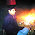I think the logic is that, from the 6 clusters you found from your code (where each cluster contains elements that a a pair of integers with common difference), D knows which cluster is the correct one (obviously, because he knows the difference, which is clearly 9 - he knows this), now if you look at the cluster in which pairwise numbers have difference 9, 2/3 elements have 32 as one of the pairwise numbers, meaning it's more likely for (32,41) or (32,23) being one of the pairs, which is why D ascertains that he 'probably' knows one of the numbers. Then, given that P already knows the difference between the numbers, he knows the pairs under consideration (because these pairs are such that they satisfy all previous conditions), which in turn allows him to say D's probability approach was incorrect, allowing D to pick the pair (64, 73) .. Apologies if I haven't articulated this well. But wow, what a brilliant problem!

13.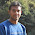why it cant be 4 ?

14.How did Sam guess the numbers based on difference

Fraction Brainteaser

Source: Sent to me by Gaurav Sinha Problem: Siddhant writes a Maths test and correctly answers 5 out of 6 Arithmetic questions and 20...